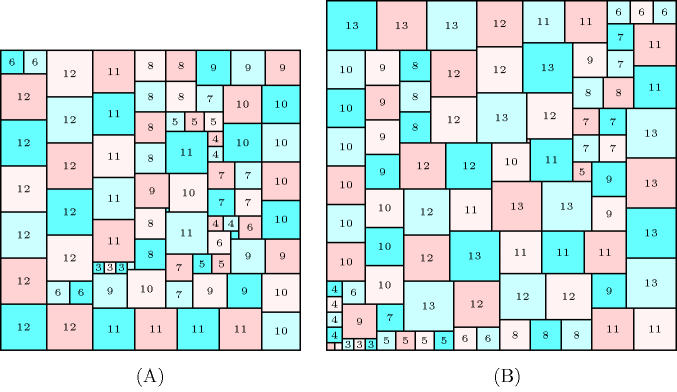### 3.7.182. Partridge

Denotes that a constraint can be used for solving the Partridge problem: the Partridge problem consists of tiling a square of size $\frac{n·\left(n+1\right)}{2}$ by $\frac{n·\left(n+1\right)}{2}$ squares of respective size

• 1 square of size 1,

• 2 squares of size 2,

•  $\cdots$ ,

• $n$ squares of size $n$.

It was initially proposed by R. Wainwright and is based on the identity $1·{1}^{2}+2·{2}^{2}+\cdots +n·{n}^{2}={\left(\frac{n·\left(n+1\right)}{2}\right)}^{2}$. The problem is described in http://mathpuzzle.com/partridge.html. Part (A) of Figure 3.7.49 gives a solution for $n=12$ found with $\mathrm{𝚐𝚎𝚘𝚜𝚝}$ [AgrenCarlssonBeldiceanuSbihiTruchetZampelli09a], while Part (B) provides a solution for $n=13$ found by S. Hougardy [Hougardy12].

##### Figure 3.7.49. (A) a solution to the Partridge problem for $n=12$, and (B) a solution for $n=13$